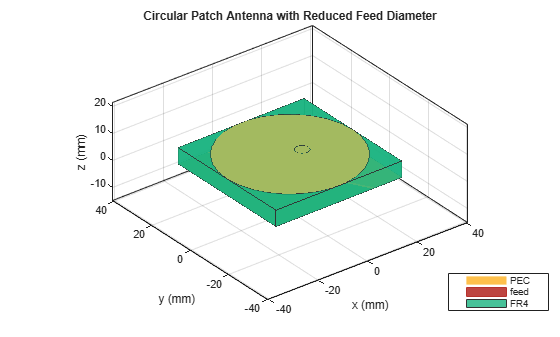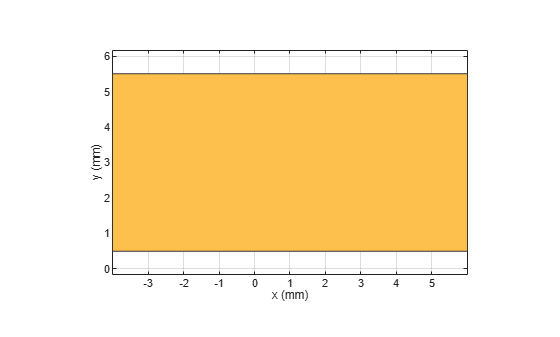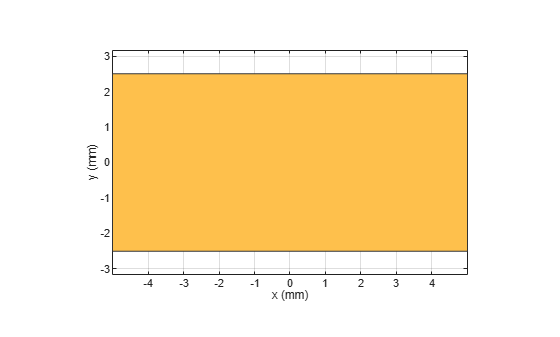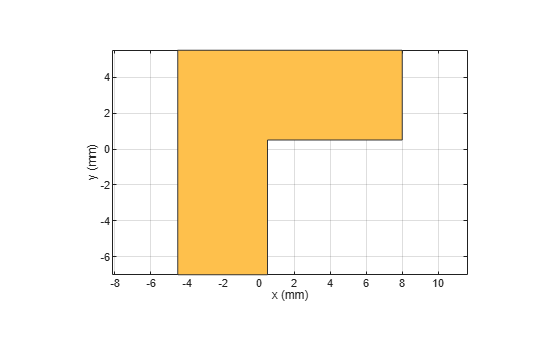Import and update Gerber files

## Description

Use the `PCBReader` object to create a printed circuit board (PCB) reader to import Gerber files and to facilitate the creation of an antenna model. A Gerber file is a set of manufacturing files used to describe a PCB antenna. A Gerber file uses an ASCII vector format to describe 2-D binary images.

## Creation

You can create a `PCBReader` object using the following methods:

• `gerberRead` — Create a `PCBReader` object with the specified Gerber and drill files.

• The `PCBReader` function described here.

### Syntax

``B = PCBReader(S)``
``B = PCBReader(Name,Value)``

### Description

example

````B = PCBReader(S)` creates a `PCBReader` object that imports multilayer PCB antenna design files described in the `stackUp` object . NoteTo translate the center of an imported symmetrical or asymmetrical polygon to `[0,0]` please use the following MATLAB® functions, `boundingbox` and `centroid`. See examples.The `PCBReader` object reads RS-274X Gerber files. It does not support RS-274D Gerber files. ```

example

````B = PCBReader(Name,Value)` sets Properties using name-value pairs. For example, `B = PCBReader('StackUp',S,'Drillfile','ant.txt')` imports the layer and drill files into the `PCBReader`. You can specify multiple name-value pairs. Enclose each property name in quotes. Properties not specified retain their default values. ```

### Input Arguments

expand all

PCB stackup definition, specified as a `stackUp` object. For more information, see `stackUp`.

Example: `S = stackUp; B = PCBReader(S)`

Example: `B = PCBReader('StackUp',S)`

## Properties

expand all

PCB stackup definition, specified as a `stackUp` object.

Example: `S = stackUp; B.StackUp = S;`

Example: `B = PCBReader('StackUp',S)`

Name of Excellon drill file, specified as a character vector or string scalar. You can specify either a DRL or a TXT file.

Example: `B.DrillFile = 'ant.drl'`

Discretization points on curved segments, specified as a positive scalar.

Example: `B.NumPointsOnCurves = 80`

## Object Functions

 `pcbStack` Single-feed or multifeed PCB antenna `shapes` Extract and modify metal layers from `PCBReader` object

## Examples

collapse all

Create a default PCB stackup definition object.

`S = stackUp;`

Set the thickness of the dielectric Air in layer 1 and layer 5 of the `stackUp` object to 0.1 mm.

```S.Layer1.Thickness = 0.1e-3; S.Layer5.Thickness = 0.1e-3;```

Import a top layer Gerber file to layer 2.

`S.Layer2 = 'antenna_design_file.gtl';`

Import a bottom layer Gerber file to layer 4.

`S.Layer4 = 'antenna_design_file.gbl';`

Create a `PCBReader` object, `B`, using the `stackUp` object, `S`.

`B = PCBReader('StackUp',S);`

Create a default PCB stackup definition object.

`s = stackUp;`

Import a top layer Gerber file to layer 2.

`s.Layer2 = 'patchMicrostripCircular_design_file.gtl';`

Create a `PCBReader` object using the `stackUp` object.

`p = PCBReader('StackUp',s);`

To update the Gerber file, convert the `PCBReader` object to a `pcbStack `object.

`p3 = pcbStack(p);`

View the `pcbStack` object.

```figure show(p3)```Update the feed diameter.

`p3.FeedDiameter = 0.005;`

View the updated `pcbStack` object.

```figure show(p3)```Plot the current distribution on the antenna at 2.4 GHz.

```figure current(p3,2.4e9)```Create a `PCBReader` object.

`B = PCBReader;`

Import a two-layer design.

```st = B.StackUp; st.Layer2 = 'UWBVivaldi.gtl'; st.Layer4 = 'UWBVivaldi.gbl'; B.StackUp = st;```

Extract shapes from the metal layers.

`S = shapes(B);`

View the top-layer Gerber file.

```figure show(S(1))```View the bottom-layer Gerber file.

```figure show(S(2))```This example will show how to translate the symmetrical polygon imported from the Gerber file to the respective co-ordinates.

Create a PCB stackup and import rectangular patch on it.

```S = stackUp; S.Layer2 = 'PatchRectangular.gtl'; S.Layer3 = dielectric('Teflon');```

Use a PCB Reader to read the polygon shape from the stackup.

```p1 = PCBReader ('StackUp',S); figure; show(p1.shapes);```Translate the shape with center (0,0) using the `centriod` function from MATLAB.

`s = p1.shapes`
```s = Polygon with properties: Name: 'mypolygon' Vertices: [4x3 double] ```
```polygon = s; [x,y] = centroid(polygon); translate(polygon,[-x, -y, 0]);```This example shows how to translate the asymmetrical polygon imported from the Gerber file to the respective co-ordinates.

Create a PCB stackup and import rectangular patch on it.

```S = stackUp; S.Layer2 = 'RightAngleBend.gtl'; S.Layer3 = dielectric('Teflon');```

Use a PCB Reader to read the polygon shape from the stackup.

```p1 = PCBReader ('StackUp',S); figure; show(p1.shapes);```Translate the shape's bottom left corner to (0,0). Use the boundingbox function from MATLAB to convert the shape to polyshape and find the upper and lower bounds of the shape.

`s = p1.shapes`
```s = Polygon with properties: Name: 'mypolygon' Vertices: [6x3 double] ```
```ver = s.Vertices(:,1:2); polygon = polyshape(ver); [xlim, ylim] = boundingbox(polygon); translate(s,[-xlim(1), -ylim(1), 0]);```## Version History

Introduced in R2020b